Lec 9 - Echelon Reduce Operations

Monday, January 23, 2012

9:29 AM

Recall:

A matrix is in reduced row echelon form (RREF) if:

1. All rows that have at least non-zero entry are above rows with only zeros.
1. The leftmost non-zero entry in a row is a 1, called a leading one.
1. The leading one in a row is to the right of the leading one in any row above it.
1. Any column containing a leading one has it as its only non-zero entry.

(Note: Every Matrix has a UNIQUE RREF)

Eg

Solve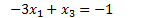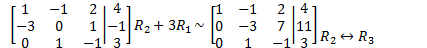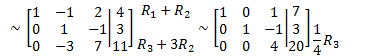Represents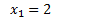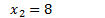Solution is:Eg

Solve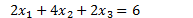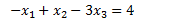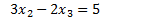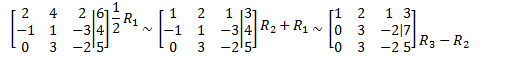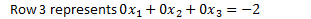Since there is no choice of variables, this system is inconsistent.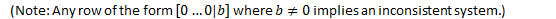Eg

Solve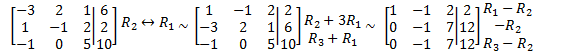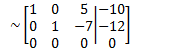Represents: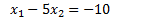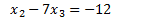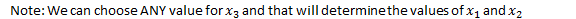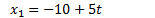The solution set of the system is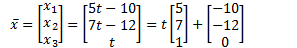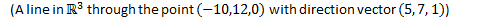Definition: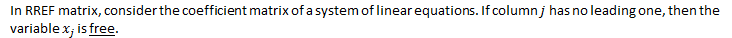Eg

Solve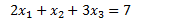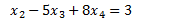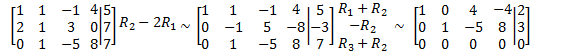Let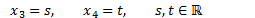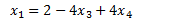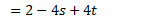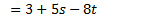The solution set is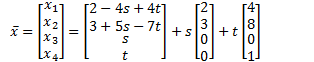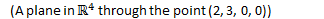EgWe must solve the system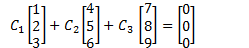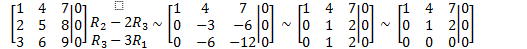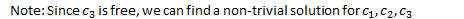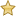Homogeneous Systems

Definition

A system of linear equations is homogeneous if the RHS contains only zeros.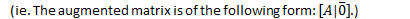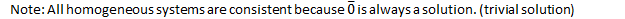Note: Performing ERPs on a homogeneous system will not change the RHS. We just perform EROs on the coefficient matrix.

Eg

Solve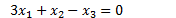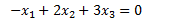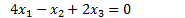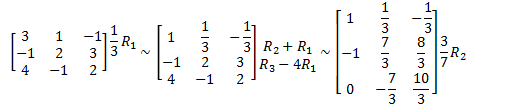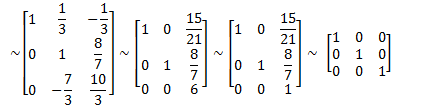Created by Tim Pei with Microsoft OneNote 2010
One place for all your notes and information